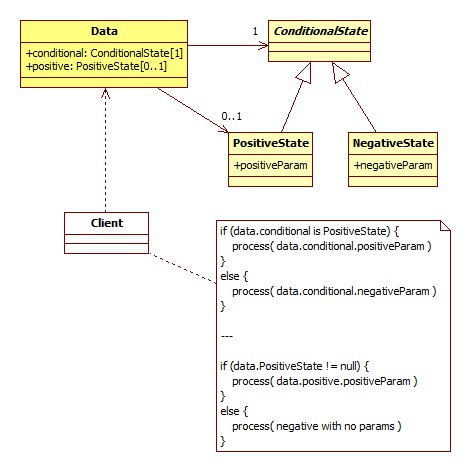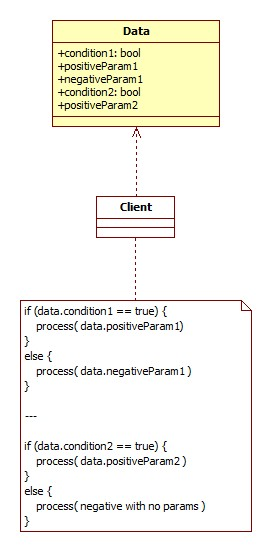Intuitive Object Models

Objects play big role in modeling software. Eventually, everything we work with is an object, including data that we need to analyze and make corresponding decisions based on it.

When we look at objects as data holders, it's very important to correctly express different states of the data as objects. This is were Intuitive Object Models come into picture.

Let's consider an example of Data class, that has following characteristics: it has two conditions attached. First condition ("conditional") is always present, and it's either in positive or in negative state. For each of positive and negative states, we are expecting additional parameter to be provided when dialing with data in the given state. Data class' second condition ("positive") can also be in positive or negative state. While for negative state, there is no additional parameter expected, for positive state, we expect to see additional parameter.

To properly solve this problem according to the "intuitive" paradigm, our Data class has two properties, one for each of the conditions. For the first ("conditional") condition, it has mandatory property that is of an abstract ConditionalState type. ConditionalState can be represented as concrete type of PositiveState or NegativeState, each providing property for additional parameter inside them. For the second ("positive") condition, Data class has optional (nullable) property that is of the PositiveState type.Above diagram demonstrates the implementation of Data class visually. It's intuitive because the following statements apply:

• Fields that are conditional and have additional parameters associated to them, can be intuitively extracted and processed. For example, "Data.conditional" is a field, which needs to provide different parameters based on its state. For positive state we can retrieve PositiveState.positiveParam parameter; for negative state we have NegativeState.negativeParam parameter. Note that the "conditional" field is required (not nullable), which means it's either in positive or in negative state at any point.
• Fields that are conditional and have additional parameters only for particular states, can be intuitively extracted and processed. For example, "Data.positive" is a field which has additional parameter requirement when it's positive, and no parameter requirement when it's negative. For that reason, this field is nullable (optional), which means the value is null when the condition is negative; when the condition becomes positive, we receive PositiveState object which exposes positiveParam for this case.

Now you can see the purpose of the term "intuitive object model". It's intuitive since we don't have to guess how the Data class should be interpreted. We just check the values of different properties and make intuitive conclusions based on them. Above diagram shows the pseudo-implementation for the possible Client class.

Anti-Intuitive Objects

The object model stops being intuitive once the above conditions mutate into these conditions:

• Fields that are conditional and have additional parameters associated with them, are presented as three fields on the data object. First field represents the condition (positive or negative), and the other two fields represent positive and negative parameters respectively. This statement is not intuitive, because the consumer of the data would have to follow particular rules to correctly interpret the data object: "if the condition is positive, take positive parameter; if the condition is negative, take negative parameter". The problem is that the three fields on the data object don't seem "intuitively" connected to each other, which makes us wonder: what if the condition is positive but the negative parameter is present instead of the positive one? is it a bug? should we process it differently? note that this kind of non-intuitive situation will never happen when following the intuitive object modeling approach.
• Fields that are conditional and have additional parameters only for particular states, are presented as two fields on the data object. First field represents the condition (positive or negative), and the second field represents the parameter for the particular condition. This model leads to questioning the situations like this: what if the condition is positive but the positive parameter is still missing? note once again that this same questionable situation cannot happen with the intuitive object modeling approach.

For completeness, I'm demonstrating the non-intuitive (wrong) approach to the object modeling below:Writing a proper Client for this kind of object model is challenging, and may even require special training or knowledge sharing sessions.